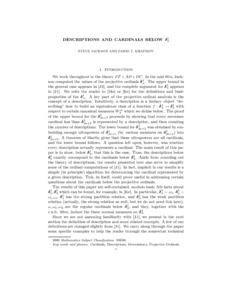# PP-2006-09: Descriptions and cardinals below $\delta^1_5$

PP-2006-09: Jackson, Steve and Khafizov, Farid (2006) Descriptions and cardinals below $\delta^1_5$. [Report]Preview Text (Full Text) PP-2006-09.text.pdf Download (218kB) | PreviewText (Abstract) PP-2006-09.abstract.txt Download (2kB)

## Abstract

We work throughout in the theory ZF+AD+DC. In the mid 80's, Jackson
computed the values of the projective ordinals \delta^1_n. The upper
bound in the general case appears in [J2], and the complete argument
for \delta^1_5 appears in [J1]. We refer the reader to [Mo] or [Ke]
for the definitions and basic properties of the \delta^1_n. A key part
of the projective ordinal analysis is the concept of a
description. Intuitively, a description is a finitary object
"describing" how to build an equivalence class of a function
f: \delta^1_3 \to \delta^1_3 with respect to certain canonical measures
W^m_3 which we define below. The proof of the upper bound for the
\delta^1_{2n+3} proceeds by showing that every successor cardinal less
than \delta^1_{2n+3} is represented by a description, and then
counting the number of descriptions. The lower bound for
\delta^1_{2n+3} was obtained by embedding enough ultrapowers of
\delta^1_{2n+1} (by various measures on \delta^1_{2n+1}) into
\delta^1_{2n+3}. A theorem of Martin gives that these ultrapowers are
all cardinals, and the lower bound follows. A question left open,
however, was whether every description actually represents a
cardinal. The main result of this paper is to show, below \delta^1_5 ,
that this is the case. Thus, the descriptions below \delta^1_5 exactly
correspond to the cardinals below \delta^1_5 . Aside from rounding out
the theory of descriptions, the results presented here also serve to
simplify some of the ordinal computations of [J1]. In fact, implicit
in our results is a simple (in principle) algorithm for determining
the cardinal represented by a given description. This, in itself,
below the projective ordinals.

The results of this paper are self-contained, modulo basic AD facts
about \delta^1_1, \delta^1_3 which can be found, for example, in
[Ke]. In particular, \delta^1_1=\omega_1, \delta^1_3=\omega_{\omega+1},
\delta^1_1 has the strong partition relation, and \delta^1_3 has the
weak partition relation (actually, the strong relation as well, but we
do not need this here). \omega, \omega_1, \omega_2 are the regular
cardinals below \delta^1_3, and they, together with the c.u.b. filter,
induce the three normal measures on \delta^1_3.

Since we are not assuming familiarity with [J1], we present in the next
section the definition of description and some related concepts. A few of our
definitions are changed slightly from [J1]. We carry along through the paper
some specific examples to help the reader through the somewhat technical
definitions. In section 4 we give an application, and present a computational
example.

Item Type: Report PP-2006-09 Prepublication (PP) Series 2006 projective ordinals; determinacy; ultrapowers Benedikt 12 Oct 2016 14:36 12 Oct 2016 14:36 https://eprints.illc.uva.nl/id/eprint/184View Item